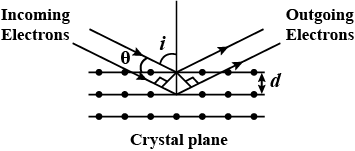Question

If a strong diffraction peak is observed when electrons are incident at and angle $$i$$ from the normal to the crystal planes with distances $$d$$ between them (see figure), de-Broglie wavelength $${\lambda _{dB}}$$ of electrons can be calculate by the relationship (n is an integer)

A
dsini=nλdBB
2dcosi=nλdBC
2dsini=nλdBD
dcosi=nλdBSolution

The correct option is B $$2\,d\,\,\cos \,\,i = n{\lambda _{dB}}$$$$2dsin\theta ={ n\lambda }_{ db }$$$$2dsin\left( 90-i \right) ={ n\lambda }_{ db }$$$$2d\left( cosi \right) ={ n\lambda }_{ db }$$Physics

Suggest Corrections0Similar questions
View MorePeople also searched for
View More